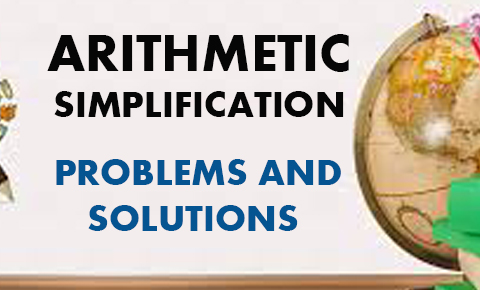Arithmetic – Simplification Problems and SolutionsArithmetic – Simplification Problems and Solutions: Arithmetic a very important topic for all type of competitive exams. Simplification  is on of the topic of Arithmetic and Quantitative aptitude. Every competitive exams has a questions on number system. This is very Read More …

Arithmetic – Square Root and Cube Roots Questions with solution and Study materialArithmetic – Square Root and Cube Roots Questions with solution and Study material: Arithmetic a very important topic for all type of competitive exams. Square root and Cube root  is on of the topic of Arithmetic and Quantitative aptitude. Every Read More …